calculus is the chain rule. This rule links together derivatives that have related variables. The chain rule is where the variable y = f(u) is a differentiable function of u and u = g(x) is a differentiable function of x. EXAMPLE: Find the derivative of"> Chain RuleCustom SearchCHAIN RULE A frequently used rule in differential calculus is the chain rule. This rule links together derivatives that have related variables. The chain rule is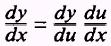where the variable y = f(u) is a differentiable function of u and u = g(x) is a differentiable function of x. EXAMPLE: Find the derivative of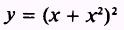SOLUTION.- Let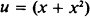and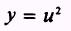Then,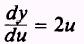andNow,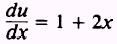and substituting into equation (1) givesbut,Therefore,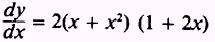EXAMPLE: Findwhereand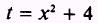SOLUTION.- By the chain ruleand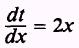Then,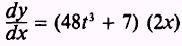and by substitution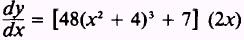PRACTICE PROBLEMS: Find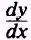in the following: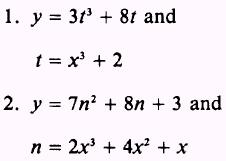ANSWERS: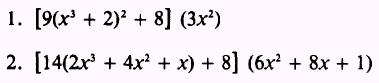Integrated Publishing, Inc. - A (SDVOSB) Service Disabled Veteran Owned Small Business